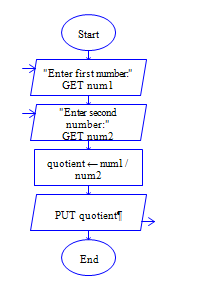# Python Division

Python program to get two integer numbers, divide both the integers and display the quotient.

Sample Input 1:

10 3

Sample Output 1:

3.3333

Sample Input 2:

29 2

Sample Output 2 :

14.5

#### Flow Chart Design#### Program or Solution

``` num1=int(input("Enter First number:")) num2=int(input("Enter second number:")) quotient=num1/num2 print(quotient) ```

#### Program Explanation

Read two inputs using input() method.

Convert it into integer by int() method.

Divide it using / operator.

It gives quotient of division operation.